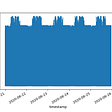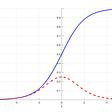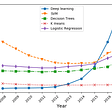# Neural nw : Inputs and targets have different numbers of samples

Hi All

I have a code , I am just checking how it works , my input matrice is :

input = [0.0600000000000000  0.00100000000000000  45  0.0508000000000000  0.0127000000000000]

and the target is a 6 by 6 matrix

so using this code bellow , I get the mentioned error : Inputs and targets have different numbers of samples ,

Error in Neural (line 17) , [net,tr] = train(net,xn_tr,yn_tr);

here is the full code :

clc clear clear all load('input.txt')%load input load ('taget.txt')%normalizing data [xn_tr,xs_tr] = mapstd(input); [yn_tr,ys_tr] = mapstd(taget); %%network net=newff(xn_tr,yn_tr,[7 7],{'tansig'},'traingda');%7 hidden tanh layer gradian descent adaptive net.trainParam.epochs =70; net.trainParam.lr = 0.05; net.trainParam.lr_inc = 1.05; %training network net.trainFcn='traingda'; [net,tr] = train(net,xn_tr,yn_tr); %randomizing initial value f weight matrix net = init(net); net.trainParam.show = NaN; u_t=mapstd('apply',x,xs_tr); %simulating output y_hat=sim(net,u_t); %plotting performance plotperform(tr) mse=mse(y-y_hat)

Matlabsolutions.com provide latest MatLab Homework Help,MatLab Assignment Help for students, engineers and researchers in Multiple Branches like ECE, EEE, CSE, Mechanical, Civil with 100% output.Matlab Code for B.E, B.Tech,M.E,M.Tech, Ph.D. Scholars with 100% privacy guaranteed. Get MATLAB projects with source code for your learning and research.

Here is a simplified example using the NEWFF example in the help and doc documentation. I omitted

1. Using an inner for loop over multiple random weight initializations and data divisions. To see those type examples search on greg Ntrials
2. Extracting the individual trn/val/tst performances via using the training record tr to obtain the corresponding indices.
% >> help newpr     % load simpleclass_dataset %      net = newpr(simpleclassInputs,simpleclassTargets,20); %      net = train(net,simpleclassInputs,simpleclassTargets); %      simpleclassOutputs = net(simpleclassInputs); close all, clear all, clc, plt = 0[ x, t ]  = simpleclass_dataset;[ I   N ] = size(x)   % [ 2 1000 ][ O N ] = size(t)    % [ 4 1000 ]trueclass = vec2ind(t);class1 = find(trueclass==1);class2 = find(trueclass==2);class3 = find(trueclass==3);class4 = find(trueclass==4);N1 = length(class1)    % 243N2 = length(class2)    % 247N3 = length(class3)    % 233N4 = length(class4)    % 277x1 =  x(:,class1);x2 =  x(:,class2);x3 =  x(:,class3);x4 =  x(:,class4);plt = plt + 1

--

--

--

## More from Technical Source

Simple! That is me, a simple person. I am passionate about knowledge and reading. That’s why I have decided to write and share a bit of my life and thoughts to.

Love podcasts or audiobooks? Learn on the go with our new app.

## Infonomics with Doug Laney## How To Determine Your Roof Slope## 5 Short Courses to Boost your Data Science Skills [Part 7]## Shabak Challenge — Data science (an attempt)## An Internship with a Freedom of Thinking## ENEM — Math score predictor## Technical Source

Simple! That is me, a simple person. I am passionate about knowledge and reading. That’s why I have decided to write and share a bit of my life and thoughts to.

## Why there are Different Cost Functions in Neural Networks## [Note] Machine Learning (Lecture 6)